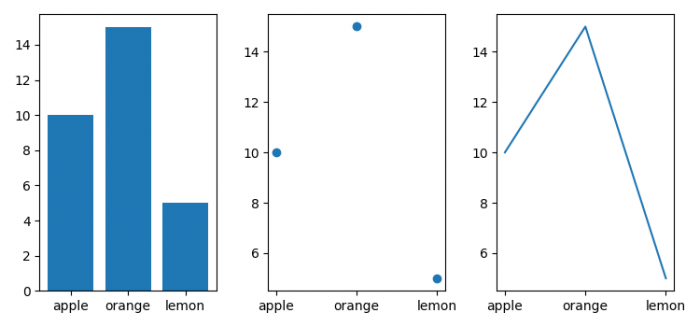# How to plot categorical variables in Matplotlib?

To plot categorical variables in Matplotlib, we can take the following steps −

• Set the figure size and adjust the padding between and around the subplots.
• Create a dictionary with some details.
• Extract the keys and values from the dictionary (Step 2).
• Create a figure and a set of subplots.
• Plot bar, scatter and plot with names and values data.
• To display the figure, use show() method.

## Example

import matplotlib.pyplot as plt

plt.rcParams["figure.figsize"] = [7.50, 3.50]
plt.rcParams["figure.autolayout"] = True

data = {'apple': 10, 'orange': 15, 'lemon': 5}
names = list(data.keys())
values = list(data.values())
fig, axs = plt.subplots(1, 3)

axs.bar(names, values)
axs.scatter(names, values)
axs.plot(names, values)

plt.show()

## Output